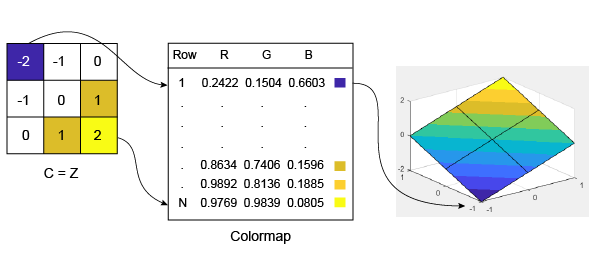曲面绘图数据与颜色图的关系

曲面与颜色图之间的关系

Surface 对象的 CData 属性包含一个索引数组 C，此数组将绘图中的特定位置与颜色图中的颜色相关联。C 与曲面 z = f(x,y) 具有如下关系：

• C 的大小与 Z 相同，其中 Z 是在曲面上每个网格点处都包含 f(x,y) 值的数组。

• C(i,j) 处的值控制曲面上网格位置 (i,j) 的颜色。

• 默认情况下，C 等于 Z，即颜色随高度而异。

• 默认情况下，C 的范围线性映射到颜色图数组中的行数。更改颜色的方向或模式

[X,Y] = meshgrid(-10:10);
Z = X + Y;
s = surf(X,Y,Z);
xlabel('X');
ylabel('Y');
zlabel('Z');C = X;
s = surf(X,Y,Z,C);
xlabel('X');
ylabel('Y');
zlabel('Z');s.CData = Y;R = sqrt(X.^2 + Y.^2) + eps;
s.CData = sin(R)./(R);